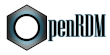OpenRDM An open-source library for reduced-density matrix-based analysis and computationTheory

# Background

Here, we use the conventional notation of multireference (MR) methods when labeling the molecular orbitals (MOs), $$\lbrace \psi\rbrace$$: the indices $$i$$, $$j$$, $$k$$, and $$l$$ denote inactive (doubly occupied) orbitals; $$t$$, $$u$$, $$v$$, and $$w$$ represent active orbitals; and $$p$$, $$q$$, $$r$$, and $$s$$ indicate general orbitals. Einstein's summation rule is implied in all expressions.

We begin by defining the non-relativistic Born-Oppenheimer (BO) electronic Hamiltonian

\begin{equation}\tag{1}\label{EQ:HAMILTONIAN} \hat{H} = h^p_q \hat{E}^p_q + \frac{1}{2} \nu^{pq}_{rs} \hat{e}^{pq}_{rs}, \end{equation}

in terms of one- and two-particle excitation operators which are defined as

\begin{gather} \hat{E}^{p}_{q} = \hat{a}^\dagger_{p_\sigma} \hat{a}_{q_\sigma} \tag{2a}\label{EQ:Epq} \\ \hat{e}^{p r}_{q s} = \hat{E}^p_q \hat{E}^r_s - \delta^q_r \hat{E}^p_s = \hat{a}^\dagger_{p_\sigma} \hat{a}^\dagger_{r_\tau} \hat{a}_{s_\tau} \hat{a}_{q_\sigma} \tag{2b}\label{EQ:epqrs} \end{gather}

In Eqs. $$\eqref{EQ:Epq}$$ and $$\eqref{EQ:epqrs}$$, the $$\hat{a}^\dagger$$ and $$\hat{a}$$ represent second-quantized creation and annihilation operators, respectively, and the Greek labels run over $$\alpha$$ and $$\beta$$ spins (the sum over which is implied). The symbol $$h^p_q = \left<\psi_p|\hat{h}|\psi_q\right>$$ represents the sum of the electron kinetic energy and electron-nucleus potential energy integrals, and $$\nu^{pq}_{rs} = \left<\psi_p \psi_q|\psi_r\psi_s\right>$$ is an element of the two-electron repulsion integral tensor. Because the electronic Hamiltonian includes up to only pair-wise interactions, the ground-state energy of a many-electron system can be expressed as an exact linear functional of the the one-electron reduced-density matrix (1-RDM) and two-electron reduced-density matrix (2-RDM)

\begin{equation}\tag{3}\label{EQ:Eel} E = {}^1D^p_q h^p_q + \frac{1}{2} {}^2D^{pq}_{rs} \nu^{pq}_{rs}. \end{equation}

Here, the 1-RDM and the 2-RDM are represented in their spin-free forms, with elements defined as

\begin{equation}\tag{4a} {}^1D^p_q = {}^1D^{p_\sigma}_{q_\sigma} = \left<\Psi|\hat{a}^\dagger_{p_\sigma} \hat{a}_{q_\sigma}|\Psi\right> \label{EQ:1RDM} \end{equation}

and

\begin{equation}\tag{4b} {}^2D^{pq}_{rs} = {}^2D^{p_\sigma q_\tau}_{r_\sigma s_\tau} = \left<\Psi|\hat{a}^\dagger_{p_\sigma} \hat{a}^\dagger_{q_\tau} \hat{a}_{s_\tau} \hat{a}_{r_\sigma}|\Psi\right> \label{EQ:2RDM}, \end{equation}

respectively. Again, the summation over the spin labels in Eqs. $$\eqref{EQ:1RDM}$$ and $$\eqref{EQ:2RDM}$$ is implied.

# Multiconfiguration Pair-Density Functional Theory

The multiconfiguration pair-density functional theory (MC-DPFT) energy expression can be written as

\begin{equation}\tag{5}\label{EQ:EMC-PDFT} E_{\text{MC-PDFT}} = 2h^i_i + h^t_u {}^1D^t_u + E_\text{H} + E_\text{xc}\left[\rho,\Pi,|\nabla\rho|,|\nabla\Pi|\right], \end{equation}

where the Hartree energy, $$E_\text{H}$$, is

\begin{equation}\tag{6}\label{EQ:EHARTREE} E_\text{H} = 2 \nu^{ij}_{ij} + 2\nu^{ti}_{ui} {}^1D^t_u + \frac{1}{2} \nu^{tv}_{uw} {}^1D^{t}_{u} {}^1D^{v}_{w} \end{equation}

The total electronic density and its gradient are defined by the 1-RDM as

\begin{equation}\label{EQ:RHO}\tag{7} \rho(\mathbf{r}) = {}^1D^p_q\ \psi^*_p(\mathbf{r}) \psi_q(\mathbf{r}), \end{equation}

and

\begin{equation}\label{EQ:DRHO}\tag{8} \nabla\rho(\mathbf{r}) = {}^1D^p_q \left[ \nabla\psi^*_p(\mathbf{r}) \psi_q(\mathbf{r}) + \psi^*_p(\mathbf{r}) \nabla\psi_q(\mathbf{r}) \right], \end{equation}

respectively. The on-top pair density (OTPD) and its gradient can similarly be defined in terms of the 2-RDM as

\begin{equation} \label{EQ:PI}\tag{9} \Pi(\mathbf{r}) = {}^2D^{pq}_{rs}\ \psi^*_p(\mathbf{r}) \psi^*_q(\mathbf{r}) \psi_r(\mathbf{r}) \psi_s(\mathbf{r}), \end{equation}

and

\begin{eqnarray} \label{EQ:DPI}\tag{10} \nabla\Pi(\mathbf{r}) = {}^2D^{pq}_{rs} &[& \nabla\psi^*_p(\mathbf{r}) \psi^*_q(\mathbf{r}) \psi_r(\mathbf{r}) \psi_s(\mathbf{r}) \nonumber \\\ &+& \psi^*_p(\mathbf{r}) \nabla\psi^*_q(\mathbf{r}) \psi_r(\mathbf{r}) \psi_s(\mathbf{r}) \nonumber \\\ &+& \psi^*_p(\mathbf{r}) \psi^*_q(\mathbf{r}) \nabla\psi_r(\mathbf{r}) \psi_s(\mathbf{r}) \nonumber \\\ &+& \psi^*_p(\mathbf{r}) \psi^*_q(\mathbf{r}) \psi_r(\mathbf{r}) \nabla\psi_s(\mathbf{r}) ~], \end{eqnarray}

respectively. Here, the 1- and 2-RDMs are obtained from an MR computation.

At this stage, we must identify a suitable OTPD functional for use in MC-PDFT. The simplest class of functionals can be derived from existing approximate exchange-correlation (XC) functionals employed within Kohn-Sham DFT by first recognizing that, for a density derived from a single Slater determinant, the spin magnetization can be expressed exactly in terms of the OTPD and the total density. [1, 2] More specifically, the spin polarization factor, $$\zeta(\mathbf{r}) = m(\mathbf{r})/\rho(\mathbf{r})$$, can be expressed as

\begin{equation} \label{EQ:ZETR}\tag{11} \zeta(\mathbf{r}) = \sqrt{1-R(\mathbf{r})}, \end{equation}

where

\begin{equation} \label{EQ:OTR}\tag{12} R(\mathbf{r}) = \frac{4~ \Pi(\mathbf{r})}{\rho^2(\mathbf{r})}. \end{equation}

The basic assumption underlying the "translated" (t) OTPD functionals proposed in Ref.  is that the spin polarization factor can be similarly defined for a density and OTPD obtained from a MR method, as

\begin{eqnarray} \label{EQ:TR}\tag{13} \zeta_{\text{tr}}(\mathbf{r}) =& \begin{cases} \sqrt{1-R(\mathbf{r})} ,& \quad R(\mathbf{r}) \leq 1 \\\ 0 ,& \quad R(\mathbf{r}) > 1 \end{cases} \end{eqnarray}

where the second case accounts for the fact that the argument of the square root can become negative for $$\rho(\mathbf{r})$$ and $$\Pi(\mathbf{r})$$ that are not derived from a single-configuration wave function. The translated OTPD functional is then defined as

\begin{equation} \label{EQ:TRE}\tag{14} E_{\text{OTPD}}\left[\rho(\mathbf{r}), \Pi(\mathbf{r}),| \nabla\rho(\mathbf{r})| \right] \equiv E_{\text{xc}}[\tilde{\rho}_\alpha(\mathbf{r}), \tilde{\rho}_\beta(\mathbf{r}), |\nabla\tilde{\rho}_\alpha(\mathbf{r})|, |\nabla\tilde{\rho}_\beta(\mathbf{r})|], \end{equation}

where the tilde refers to translated densities and their gradients, given by [3,4]

\begin{equation} \label{EQ:TRHO}\tag{15} \tilde{\rho}_\sigma(\mathbf{r}) = \frac{\rho(\mathbf{r})}{2} \left(1 + c_\sigma \zeta_{\text{tr}}(\mathbf{r})\right), \end{equation}

and

\begin{equation}\tag{16} \nabla\tilde{\rho}_\sigma(\mathbf{r}) = \frac{\nabla\rho(\mathbf{r})}{2} \left(1 + c_\sigma \zeta_{\text{tr}}(\mathbf{r})\right), \end{equation}

respectively. Here, $$c_\sigma$$ = 1~(-1) when $$\sigma = \alpha$$ ( $$\beta$$).

It is important to note that, in deriving the translated OTPD functional expression in Eq. $$\eqref{EQ:TRE}$$, no dependence on $$\nabla\Pi(\mathbf{r})$$ is assumed. A scheme in which the OTPD functional depends explicitly upon $$\nabla\Pi(\mathbf{r})$$ has also been proposed. The corresponding "fully-translated" (ft) functionals are defined as

\begin{equation} \label{EQ:FTRE}\tag{17} E_{\text{OTPD}}\left[\rho(\mathbf{r}), \Pi(\mathbf{r}), |\nabla\rho(\mathbf{r})|,|\nabla\Pi(\mathbf{r}) \right|] \equiv E_{\text{xc}}[\tilde{\rho}_\alpha(\mathbf{r}), \tilde{\rho}_\beta(\mathbf{r}), |\nabla\tilde{\rho}_\alpha(\mathbf{r})|, |\nabla\tilde{\rho}_\beta(\mathbf{r})|] \end{equation}